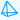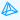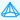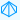Math Calculator

## Pyramid CalculatorRegular Triangular Pyramid Area of Base :[ ½as ] Surface Area of Pyramid :[ ½as + (3/2)sl ] Volume of Pyramid :[ (1/6)abh ] Enter the Apothem Length = Enter the Side Length = Enter the Slant height = Enter the Height = Area of Base = Surface Area of Pyramid = Volume of Pyramid =Regular Square Pyramid Area of Base :[ s² ] Surface Area of Pyramid :[ s² + 2sl ] Volume of Pyramid :[ (1/3)b²h ] Enter the Side Length = Enter the Slant height = Enter the Height = Area of Base = Surface Area of Pyramid = Volume of Pyramid =Regular Pentagonal Pyramid Area of Base :[ (5/2)as ] Surface Area of Pyramid :[ (5/2)as + (5/2)sl ] Volume of Pyramid :[ (5/6)abh ] Enter the Apothem Length= Enter the Side Length= Enter the Slant height = Enter the Height = Area of Base = Surface Area of Pyramid = Volume of Pyramid =Regular Hexagonal Pyramid Area of Base :[ (6/2)as ] Surface Area of Pyramid :[ 3as + 3sl ] Volume of Pyramid :[ abh ] Enter the Apothem Length= Enter the Side Length= Enter the Slant height = Enter the Height = Area of Base = Surface Area of Pyramid = Volume of Pyramid =